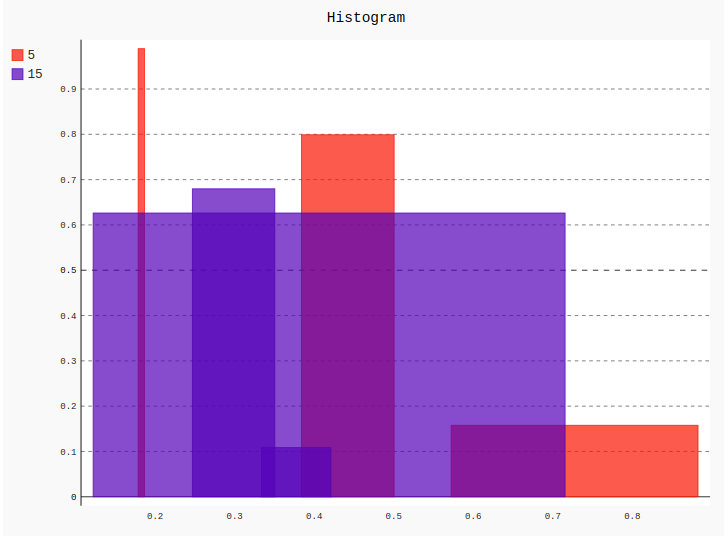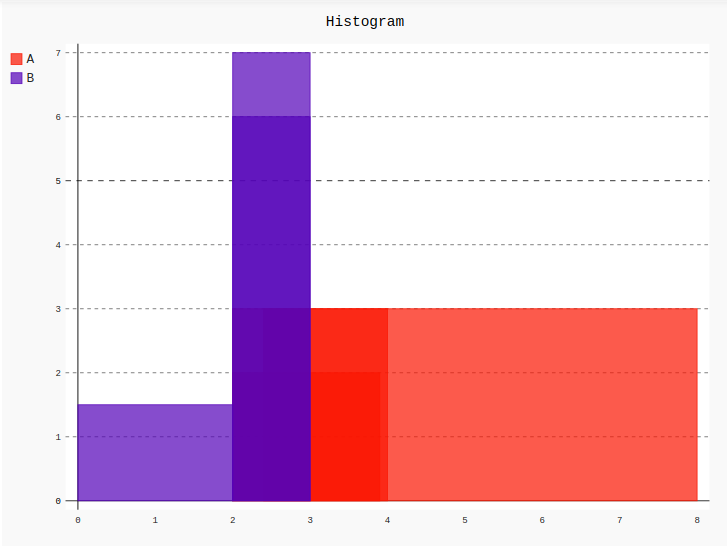# Histogram in Pygal

Pygal is a Python module that is mainly used to build SVG (Scalar Vector Graphics) graphs and charts. SVG is a vector-based graphics in the XML format that can be edited in any editor. Pygal can create graphs with minimal lines of code that can be easy to understand and write.

## Histogram

The histogram is a graphical display of data using bars of different heights, it is similar to bar chart but the histogram presents the number of groups into ranges and presents them in segment columns. Histogram chart is only used for plotting the frequency of score occurrences in a continuous data set that has been divided into classes, called bins. Histogram are those type of special bars which take 3 values for a bar:

• The ordinate height
• The abscissa start
• The abscissa end

Syntax:

`hist = pygal.Histogram()`

Example 1:

 `# importing pygal ` `import` `pygal ` `import` `numpy ` ` `  ` `  `# creating the chart object ` `histogram ``=` `pygal.Histogram() ` ` `  `# naming the title ` `histogram.title ``=` `'Stacked Bar Chart'`         ` `  `# Random data ` `for` `i ``in` `range``(``5``, ``20``, ``10``): ` `    ``data ``=` `list``(``zip``(numpy.random.rand(i), ` `                    ``numpy.random.rand(``3``),  ` `                    ``numpy.random.rand(i))) ` `    ``histogram.add(``str``(i), data) ` ` `  `histogram `

Output:Example 2:

 `# importing pygal ` `import` `pygal ` `import` `numpy ` ` `  ` `  `# creating the chart object ` `histogram ``=` `pygal.Histogram() ` ` `  `# naming the title ` `histogram.title ``=` `'Histogram'`         ` `  `histogram.add(``'A'``, [(``2``, ``2``, ``3.9``), (``3``, ``2``, ``8``), (``3``, ``4``, ``2.4``)]) ` `histogram.add(``'B'``, [(``1.5``, ``0``, ``2``), (``6``, ``2``, ``3``), (``7``, ``3``, ``2``)]) ` ` `  `histogram `

Output:My Personal Notes arrow_drop_upIf you like GeeksforGeeks and would like to contribute, you can also write an article using contribute.geeksforgeeks.org or mail your article to contribute@geeksforgeeks.org. See your article appearing on the GeeksforGeeks main page and help other Geeks.

Please Improve this article if you find anything incorrect by clicking on the "Improve Article" button below.

Article Tags :

Be the First to upvote.

Please write to us at contribute@geeksforgeeks.org to report any issue with the above content.